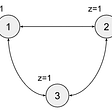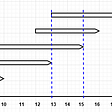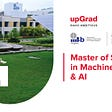# Decidability Problem of Turing Machines

To be precise about the notion of what we mean by “computational problems” and what it means for a Turing machine to “solve” a problem, we define problems in terms of languages, which correspond to decision problems with a yes/no answer. We define two notions of “solving” a problem specified by a language L. The first of these definitions (“deciding L”) corresponds to what we usually mean when we speak of solving a computational problem, i.e. terminating and outputting a correct yes/no answer. The second definition (“accepting L”) is a “one-sided” definition: if the answer is “yes”, the machine must halt and provide this answer after a finite amount of time; if the answer is “no”, the machine need not ever halt and provide this answer. Finally, we give some definitions that apply to computational problems where the goal is to output a string rather than just a simple yes/no answer. Let Σ0 = Σ \ {►, ⨿}. A language is any set of strings L ⊆ Σ∗ 0. Suppose M is a Turing machine and L is a language. M decides L if every computation of M halts in the “yes” or “no” state, and L is the set of strings occurring in starting configurations that lead to the “yes” state. L is decidable if there is a machine M that decides L. M accepts L if L is the set of strings occurring in starting configurations that lead M to halt in the “yes” state. L is recursively enumerable if there is a machine M that accepts L. M computes a given function f : Σ0∗ → Σ0* if every computation of M halts, and for all x ∈ Σ0*, the computation with starting configuration ( x , s, 0) ends in configuration ( ►f(x) ⨿· · ·⨿ , halt, 0), where ⨿· · ·⨿ denotes any sequence of one or more repetitions of the symbol ⨿. f is a computable function if there is a Turing machine M that computes f.

# Halting Problem if Turing Machines

The halting problem is the problem of deciding whether a given Turing machine halts when presented with a given input. In other words, it is the language H defined by:

H = {M; x | M is a valid Turing machine description, and M halts on input x}

x ∈ L(MD) ⇐⇒ MD(x) = yes ⇐⇒ MD(x) 6= no ⇐⇒ x 6∈ L(x) ⇐⇒ x ∈ D

So we have proved that L(MD) = D, i.e. MD is a Turing machine that accepts D, as claimed.

# Rice’s Theorem

In particular, the question of whether a given Turing machine halts on a given input is not decidable. Unfortunately, the situation is much worse than this! Our next theorem shows that essentially any non-trivial property of Turing machines is undecidable. (Actually, this is an overstatement; the theorem will show that any non-trivial property of the languages accepted by Turing machines is undecidable. Non-trivial properties of the Turing machine itself for example does it run for more than 100 steps when presented with the input string ►⨿ may be decidable.) Language L ⊆ Σ∗ 0 is a Turing machine I/O property if it is the case that for all pairs x, y ∈ Σ∗ such that L(x) = L(y), we have x ∈ L ⇔ y ∈ L. We say that L is non-trivial if both of the sets L and L = Σ0* \ L are nonempty. Rice’s Theorem stated that If L is a non-trivial Turing machine I/O property, then L is undecidable.

Proof: Consider any x1 such that L(x1) = ∅. We can assume without loss of generality that x1

L. The reason is that a language is decidable if and only if its complement is decidable. Thus, if x1 ∈ L then we can replace L with L` and continue with the rest of proof. In the end we will have proven that L` is undecidable from which it follows that L is also undecidable. Since L is non-trivial, there is also a string x0 ∈ L. This x0 must be a description of a Turing machine M0. (If x0 were not a Turing machine description, then it would be the case that L(x0) = ∅ = L(x1), and hence that x0 ∉ L since x1 ∉ L and L is a Turing machine I/O property.) Given a Turing machine ML that decides L we will construct a Turing machine MH that decides the halting problem, in contradiction to Theorem Halting Theorem defined in section 6. The construction of MH is as follows. On input M; x, it transforms the pair M; x into the description of another Turing machine M∗, and then it feeds this description into ML. The definition of M∗ is a little tricky. On input y, machine M∗ does the following. First it runs M on input x, without overwriting the string y. If M ever halts, then instead of halting M∗ enters the second phase of its execution, which consists of running M0 on y. (Recall that M0 is a Turing machine whose description x0 belongs to L.) If M never halts, then M∗ also never halts. This completes the construction of MH. To recap, when MH is given an input M; x it first transforms the pair M; x into the description of a related Turing machine M∗, then it runs ML on the input consisting of the description of M∗ and it outputs the same answer that ML outputs. There are two things we still have to prove. The function that takes the string M; x and outputs the description of M∗ is a computable function, i.e. there is a Turing machine that can transform M; x into the description of M∗. Assuming ML decides L, then MH decides the halting problem.

# Non-Deterministic Turing Machines

Turing machines we have been discussing have all been deterministic, meaning that there is only one valid computation starting from any given input. Nondeterministic Turing machines are defined in the same way as their deterministic counterparts, except that instead of a transition function associating one and only one (p, τ, d) to each (state, symbol) pair (q, σ), there is a transition relation (which we will again denote by δ) consisting of any number of ordered 5-tuples (q, σ, p, τ, d). If (q, σ, p, τ, d) belongs to δ, it means that when observing symbol σ in state q, one of the allowed behaviors of the nondeterministic machine is to enter state p, write τ, and move in direction d. If a nondeterministic machine has more than one allowed behavior in a given configuration, it is allowed to choose one of them arbitrarily. Thus, the relation (x, q, k) →M (x`, q`, k`) is interpreted to mean that configuration (x`, q`, k`) is obtained from (x, q, k) by applying any one of the allowed rules (q, xk, p, τ, d) associated to (state, symbol) pair (q, xk) in the transition relation δ. Given this interpretation of the relation →M. If M is a nondeterministic Turing machine and x ∈ Σ∗0 is a string, we say that M accepts x if there exists a computation of M starting with input ► x ⨿ that ends by halting in the “yes” state. The set of all strings accepted by M is denoted by L(M).

This interpretation of “M accepts x” illustrates the big advantage of nondeterministic Turing machines over deterministic ones. In effect, a nondeterministic Turing machine is able to try out many possible computations in parallel and to accept its input if any one of these computations accepts it. No physical computer could ever aspire to this sort of unbounded parallelism, and thus nondeterministic Turing machines are, in some sense, a pure abstraction that does not correspond to any physically realizable computer. (This is in contrast to deterministic Turing machines, which are intended as a model of physically realizable computation, despite the fact that they make idealized assumptions (such as an infinitely long tape) that are intended only as approximations to the reality of computers having a finite but effectively unlimited amount of storage.) However, nondeterminism is a useful abstraction in computer science for a couple of reasons. Turing machine that accepts language L then there is also a deterministic Turing machine that accepts L. Thus, if one ignores running time, nondeterministic Turing machines have no more power than deterministic ones. The question of whether nondeterministic computation can be simulated deterministically with only a polynomial increase in running time is the P vs. NP question, perhaps the deepest open question in computer science. Nondeterminism can function as a useful abstraction for computation with a non-trusted external source of advice: the nondeterministic machine’s transitions are guided by the advice from the external source, but it remains in control of the decision whether to accept its input or not. One useful way of looking at nondeterministic computation is by relating it to the notion of a verifier for a language. Let Σ be a language not containing the symbol ‘;’. A verifier for a language L ⊆ Σ∗ 0 is a deterministic Turing machine with alphabet Σ ∪ {;}, such that L = {x | ∃ y s.t. V(x; y) = yes}. We say that V is a polynomial-time verifier for L if V is a verifier for L and there exists a polynomial function p such that for all x ∈ L there exists a y such that V(x; y) outputs “yes” after at most p(| x|) steps. (Note that if any such y exists, then there is one such y satisfying | y| ≤ p(| x|), since the running time bound prevents V from reading any symbols of y beyond the first p(| x|) symbols.)

--

--

--

## More from Ammar Khalid Shamsi

I have no special talents. I am only Passionately Curious…

Love podcasts or audiobooks? Learn on the go with our new app.

## Web Development During COVID-19## Building Better Concurrency, Finite State Processes, and Modeling Processes## Creating a Window in Unity## Coding Crowd+ ✨ Clean ✨## Story of clean code## Serverless and its benefits## Distributed Systems— CAP, simplified## How to Make Your Own Python Package## Ammar Khalid Shamsi

I have no special talents. I am only Passionately Curious…

## Solving Intersections by Marzullo’s Way## Goodbye Earth and on to the “Metaverse”. Beam me up Scotty!## What are the differences between centralized learning (in monolithic systems) and decentralized…## My take on AI/ML course with Upgrad and IIIT-B